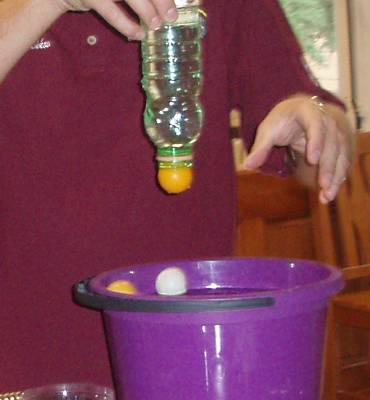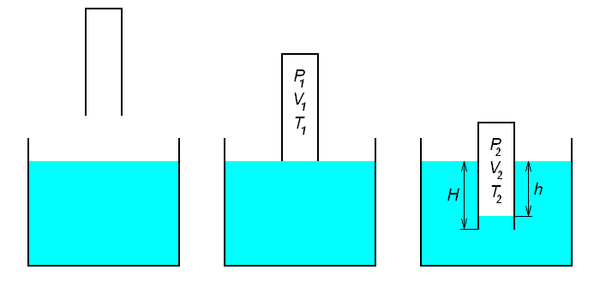### O příspěvku

• SŠ/VŠ

#### Pomůcky

• S opravdu jednoduchými pomůckami
• S jednoduchými pomůckami

## Atmospheric pressure in low-cost demonstrations and measurements

Kazachkov A., Salinas A.

### Introduction

In a Physics class, lab work is essential. Experimental physics projects are an opportunity for educators to make more interesting Physics to students. Experiments that involve knowledge of many parts of physics are important to develop their creativity and to make knowledge more significant for students (significative learning) . The laboratory of Physics is also the place where students get the opportunity to do physics. It is in the laboratory that students learn to practice the activities of scientists – asking questions, performing procedures, collecting data, analyzing data, answering questions, and thinking of new questions to explore. Physics teachers should exploit the laboratory projects to teach in a more interesting manner this subject. It is also important to do the experimental work with materials easy to find, otherwise students get the impression that science can be performed only with sophisticated equipment. On the other hand, there are schools in some developing countries which do not have equipped laboratories and no computers at all. Students in those schools do not have the opportunity to manipulate modern technology used today for physics experiments. Teachers in these schools need to learn simple and easy ways to prove physical facts of nature to their students.

We present here two simple experiments to measure the atmospheric pressure with the materials easy to find and no cost at all. These experiments can be performed by high school students and permit them to diminish the error by modifying the experiment in an easy manner.

### Measuring Patm using a ping pong ball and water containers

A precise measurement of atmospheric pressure is based on the well-known demonstration in which a cup of water is covered with a pressed piece of cardboard and inverted . When the cardboard is released, no water spills out. The explanation is straightforward. Forced by gravity to slip downward slightly, the column of water acts like a piston, reducing the air pressure inside the cup until equilibrium is achieved. The slip distance is small (typically less than a millimeter) and so the cardboard is displaced only very slightly from the glass. The surface tension of the water is sufficient to prevent it from seeping out through the tiny gap. The demonstration works reliably with a short cylindrical container but can often fails for a tall partly filled cylinder or bottle [3, 4] because the slip distance depends on the volume of water in the container. We modify the demo using a ping pong ball instead of the flat card as shown in Fig. 1, which makes the experiment more simple and measuring.Figure 1. The use of a ping pong ball simplifies the experiment and makes it measuring.

We start with a glass bottle that is partly full of water and hold a ball firmly against the top as we invert the bottle (Fig 2(a)). If the ball is simply released, the column of water would slip so far down that it would push the ball away from the opening and all the water would spill out. Instead, we hold the cardboard loosely and allow water to gradually drip out of the bottle into a cup below (Fig. 2(b)). The dripping continues until the difference in the air pressure inside (P) and outside (atmospheric, Patm) balances the pressure ρgh that the water column exerts onto the ball (here h is the height of the column, ρ is density of water or whatever liquid is used, and g is the freefall acceleration). At that point the dripping ceases (Fig. 1(c)). The equilibrium condition is $\tag{1} P + \rho gh = P_{atm} .$Figure 2. Schematic representation of the experiment: (a) initial position; (b) water is gradually released; (c) equilibrium is achieved .

While the water is dripping out of the bottle, it is important to keep any outside air from leaking in. Heating by the hand holding the bottle should also be prevented. If these conditions are satisfied, the mass and temperature of the air inside the bottle remains unchanged during the process, and we can use Boyle’s law to write $\tag{2} P_{atm}V = P(V + \Delta V) .$

Here V is the initial volume of air inside the inverted bottle and ∆V is the increase in the air’s volume, which exactly equals the volume of the water that dripped into the cup below.

Solving Eq. (1) for P and substituting into Eq. (2) gives, after a bit of rearranging, $\tag{3} P_{atm} = \rho gh(1 + V/\Delta V).$

Using the known values of ρ and g, along with the easily measured values of V and ∆V, Patm can now be determined.

### Alexander´s diving bell measuring Patm

Another simple hydrostatic experiment to measure the atmospheric pressure is again an application of students’ knowledge of gas laws . They lower a straight transparent test tube open end down into a tank of water. Due to the pressure of the captured air, water level inside the tube never reaches that of the tank. To determine the atmospheric pressure, students must measure the depth of the tube’s lowering the water level inside the tube; the local value of the acceleration of gravity must be known. We suggest attaching the tube to a bigger air-filled container, which dramatically increases accuracy of measurements and opens a variety of experimental possibilities .

Figure 3 and 4 show the experimental set up required for this experiment .Figure 3.The tube is submerged with the open end down into a water tank. By measuring h and H, it is possible to determine Patm.

From Figure 3 and from the Clapeyron-Mendeleev equation, we get $\tag{4} \frac{P_1 V_1}{T_1} = \frac{P_2 V_2}{T_2}.$

If the temperatures inside and outside the tube are equal T1 = T2, $\tag{5} P_1 V_1 = P_2 V_2.$

But also P1 = Patm , the atmospheric pressure and thus P2 = Patm + ρgh.

From the last two equations we get $\tag{6} \rho g h = \frac{P_{atm}(V_1 - V_2)}{V_2} = \frac{P_{atm}\Delta V}{V_2} .$

From this equation we finally obtain $\tag{7} P_{atm} = \frac{\rho g h V_2}{\Delta V}.$

Or for a series of measurements with varied H, using (6), we can plot ρgh vs $$\frac{P_{atm}\Delta V}{V_2}$$. The graph is a straight line with the slope being Patm.

Crucial for the method to be practical is the right choice of the apparatus’ parameters (Fig. 4), in particular, of the ratio between V1 and H. Otherwise, either h or the H – h difference are too small to measure in class conditions.Figure 4. The volume of the air V1 is increased by attaching a large container (left), or by taking a long enough hose (right). H – h is dramatically increased with this adaptation.

### Conclusions

The presented experiments were performed by a variety of students, ranging from high school to university in USA and Ukraine for the case of the water containers with a ping pong ball  and Mexico and Ukraine for the modified Alexander´s diving bell experiment, where in fact students were able to measure the acceleration of gravity assuming the atmospheric pressure was known . Those experiments allow students to develop their creativity by making modifications in order to make the experiment more precise. Students were able to apply their knowledge and to actually experience scientific work. These experiments also motivate students and, as assignment projects, they encourage new lines of thinking. In all cases, students exhibited strong engagement and high interest suggesting new ideas on how to improve the results.

With those simple experiments, students also realize that in order to experience science it is not necessary to have expensive equipment. They can use objects from everyday life which have no cost at all. These activities also encourage educators and students from schools with poor equipment in their laboratories to experiment and obtain important physical constants.

It is hoped that educators apply these experiments in their lab work with their students and also suggest new ways to improve the accuracy of the measurements. Details and measuring results can be found in  and .

### Bibliography

 Salinas, A., Didactic Proposal for Projects in the Physics Lab. Work presented at the VI Congreso Internacional Didáctica de las Ciencias, La Havana, Cuba, March 15-19, 2010. http://www.didacien.rimed.cu/Didacticas%20de%20las%20Ciencias/Simposios/Simposio6/Trabajos/F%EDs%20006.pdf. Consulted: August, 2012.

 A. Kazachkov, D. Kryuchkov, C. Willis and J.C. Moore, An Atmospheric Pressure Ping Pong Ballometer,  Phys. Teach. 44, 492 (2006).

 J.Walker, The Flying Circus of Physics (Wiley, New York, 1977), p. 79.

 R.Ehrlich, Turning the World Inside Out and 174 Other Simple Physics Demonstrations (Princeton University Press, Princeton, NJ, 1990), p. 102.

 M. Quiroga, S. Martínez and S. Otranto, A Measurement of g using Alexander´s Diving Bell, ,  Phys. Teach. 48, 386-387, (2010).

 A. Kazachkov, A. Salinas and V. Kostyukov, Measuring g: an Inexhaustible Source of Instruction and Creativity, Lat. Am. J. Phys. Educ. Vol. 6, Suppl. I, June 2012.